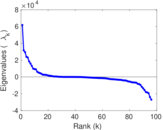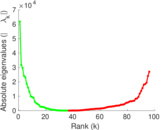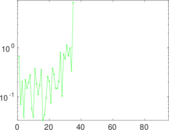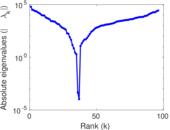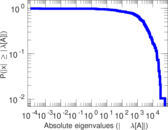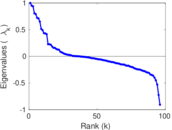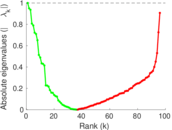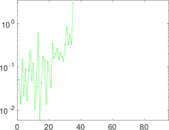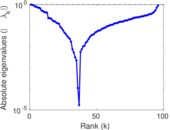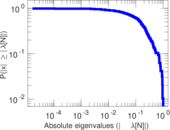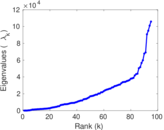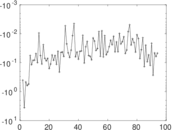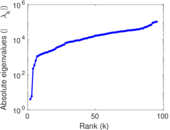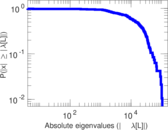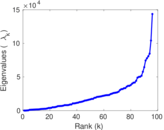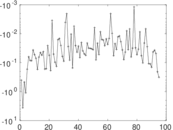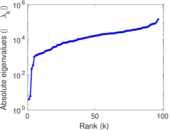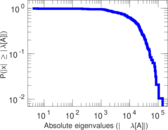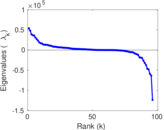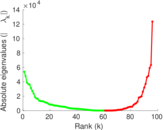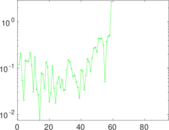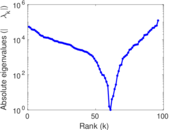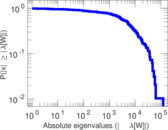# Reality Mining

This undirected network contains human contact data among 100 students of the Massachusetts Institute of Technology (MIT), collected by the Reality Mining experiment performed in 2004 as part of the Reality Commons project. The data was collected over 9 months using 100 mobile phones. A node represents a person; an edge indicates that the corresponding nodes had physical contact.

 Code `RM` Internal name `mit` Name Reality Mining Data source http://realitycommons.media.mit.edu/realitymining.html AvailabilityDataset is available for download Consistency checkDataset passed all tests Category Human contact network Node meaning Person Edge meaning Contact Network format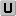Unipartite, undirected Edge typeUnweighted, multiple edges Temporal dataEdges are annotated with timestamps LoopsDoes not contain loops

## Statistics

 Size n = 96 Volume m = 1,086,404 Unique edge count m̿ = 2,539 Wedge count s = 149,335 Claw count z = 3,073,975 Cross count x = 48,933,259 Triangle count t = 36,108 Square count q = 1,534,878 4-Tour count T4 = 12,881,442 Maximum degree dmax = 98,257 Average degree d = 22,633.4 Fill p = 0.556 798 Average edge multiplicity m̃ = 427.887 Size of LCC N = 96 Diameter δ = 3 50-Percentile effective diameter δ0.5 = 0.795 737 90-Percentile effective diameter δ0.9 = 1.733 01 Median distance δM = 1 Mean distance δm = 1.363 27 Relative edge distribution entropy Her = 0.982 596 Power law exponent γ = 1.317 13 Tail power law exponent γt = 2.611 00 Tail power law exponent with p γ3 = 2.611 00 p-value p = 0.000 00 Degree assortativity ρ = −0.055 486 2 Degree assortativity p-value pρ = 7.620 70 × 10−5 Clustering coefficient c = 0.725 376 Spectral norm α = 61,801.9 Algebraic connectivity a = 4.041 03 Spectral separation |λ1[A] / λ2[A]| = 1.950 75 Non-bipartivity bA = 0.563 644 Normalized non-bipartivity bN = 0.783 795 Algebraic non-bipartivity χ = 1.973 50 Spectral bipartite frustration bK = 0.009 327 28 Controllability C = 1 Relative controllability Cr = 0.010 416 7

## Plots

### Fruchterman–Reingold graph drawing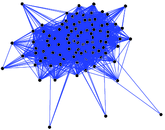### Degree distribution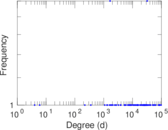### Cumulative degree distribution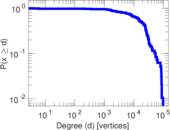### Lorenz curve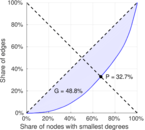### Spectral distribution of the adjacency matrix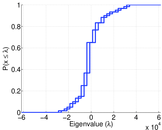### Spectral distribution of the normalized adjacency matrix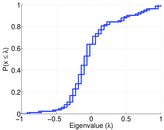### Spectral distribution of the Laplacian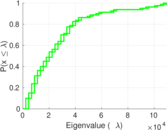### Spectral graph drawing based on the adjacency matrix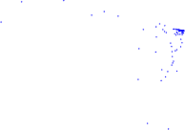### Spectral graph drawing based on the Laplacian### Spectral graph drawing based on the normalized adjacency matrix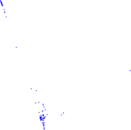### Degree assortativity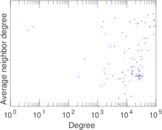### Zipf plot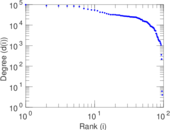### Hop distribution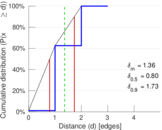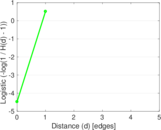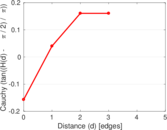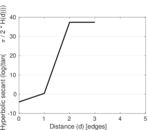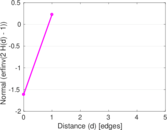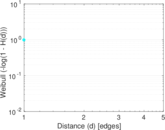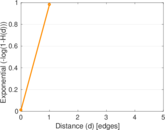### Double Laplacian graph drawing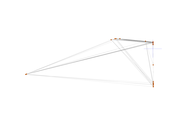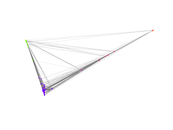### Delaunay graph drawing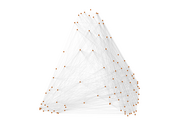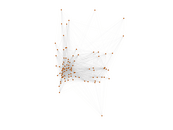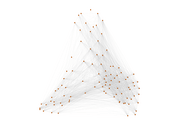### Edge weight/multiplicity distribution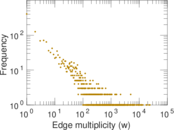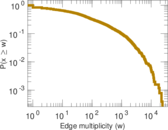### Clustering coefficient distribution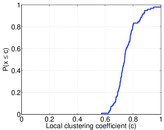### Average neighbor degree distribution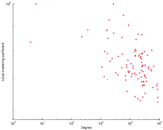### Temporal distribution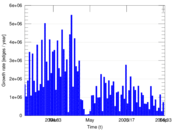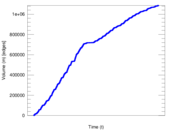### Temporal hop distribution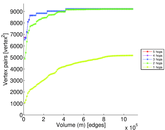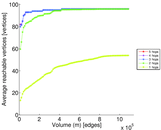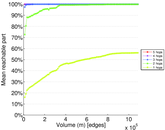### Diameter/density evolution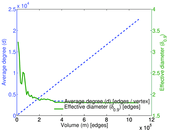### Inter-event distribution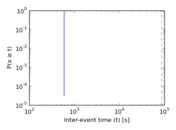### Node-level inter-event distribution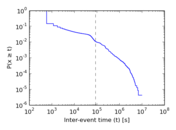### Matrix decompositions plots